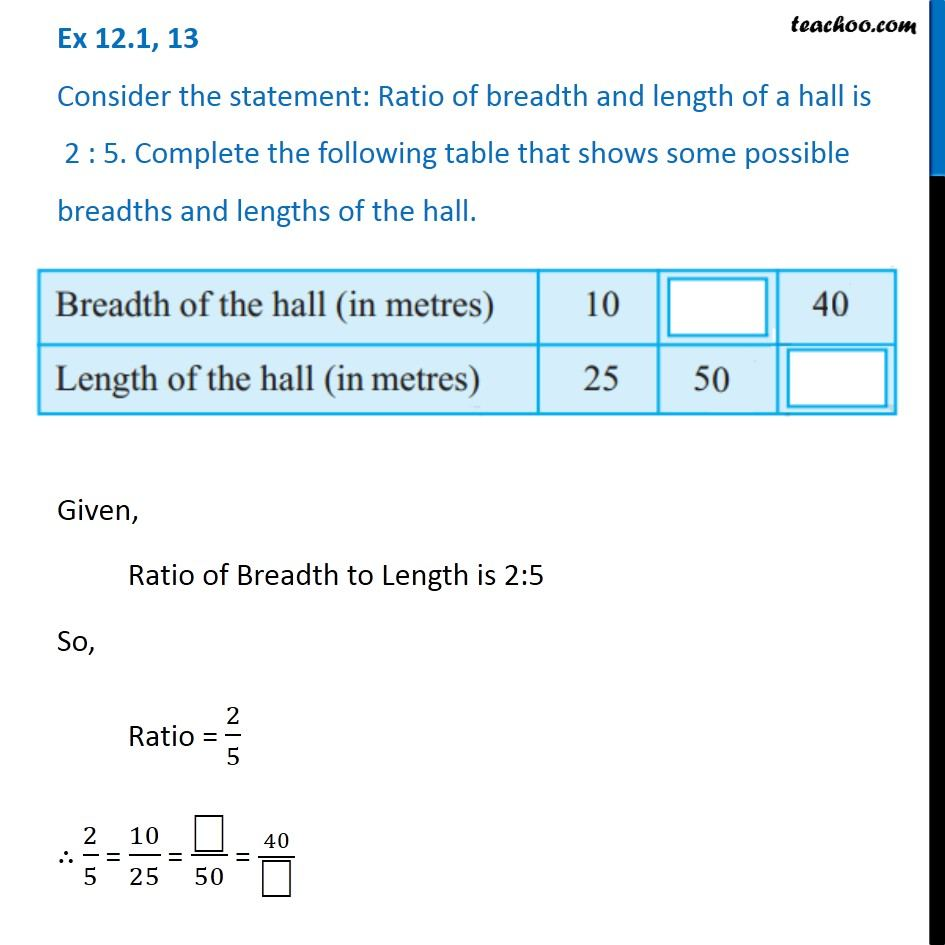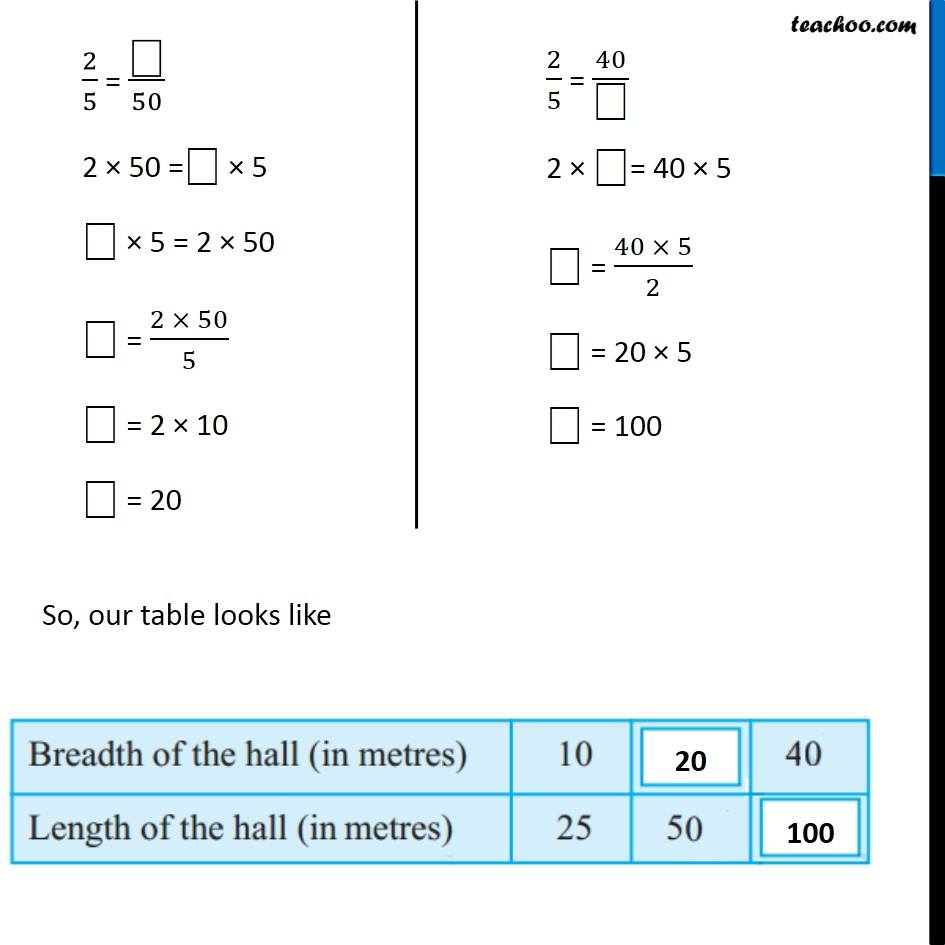Ex 12.1

Chapter 12 Class 6 Ratio and Proportion
Serial order wiseLearn in your speed, with individual attention - Teachoo Maths 1-on-1 Class

### Transcript

Ex 12.1, 13 Consider the statement: Ratio of breadth and length of a hall is 2 : 5. Complete the following table that shows some possible breadths and lengths of the hall.Given, Ratio of Breadth to Length is 2:5 So, Ratio = 2/5 ∴ 2/5 = 10/25 = "⎕" /50 = 40/"⎕" 2/5 = "⎕" /50 2 × 50 ="⎕" × 5 "⎕" × 5 = 2 × 50 "⎕" = (2 × 50)/5 "⎕" = 2 × 10 "⎕" = 20 2/5 = 40/"⎕" 2 × "⎕"= 40 × 5 "⎕" = (40 × 5)/2 "⎕" = 20 × 5 "⎕" = 100 2/5 = 40/"⎕" 2 × "⎕"= 40 × 5 "⎕" = (40 × 5)/2 "⎕" = 20 × 5 "⎕" = 100